### Symmetry - Solutions

CBSE Class –VII Mathematics

NCERT Solutions
Chapter 14 Symmetry (Ex. 14.1)

Question 1. Copy the figures with punched holes and find the axes of symmetry for the following:
(a)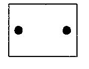(b)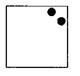(c)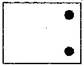(d)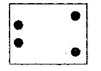(e)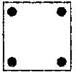(f)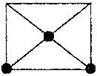(g)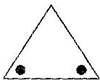(h)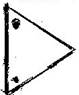(i)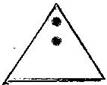(j)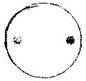(k)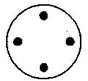(l)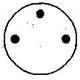S.No. Punched holed figures The axes of symmetry (a)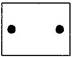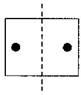(rectangle) (b)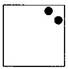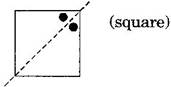(c)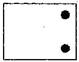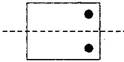(d)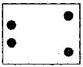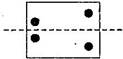(e)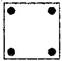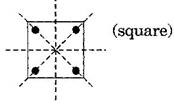(f)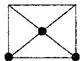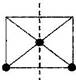(g)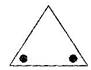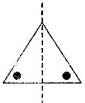(h)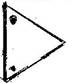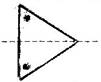(i)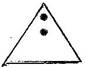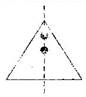(j)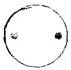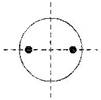(k)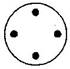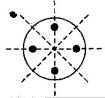(l)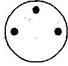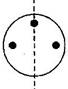Question 2. Given the line(s) of symmetry, find the other hole(s):

 S.No. Line(s) of symmetry Other holes on figures (a)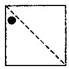(b)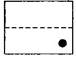(c)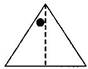(d)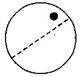(e)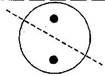Ans.

 S.No. Line(s) of symmetry Other holes on figures (a)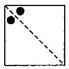(b)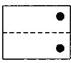(c)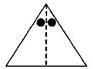(d)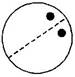(e)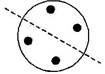Question 3. In the following figures, the mirror line (i.e., the line of symmetry) is given as a dotted line. Complete each figure performing reflection in the dotted (mirror) line. (You might perhaps place a mirror along the dotted line and look into the mirror for the image).

Are you able to recall the name of the figure you complete?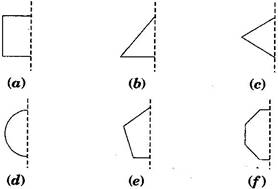S.No. Question figures Complete figures Names of the figure (a)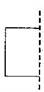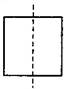Square (b)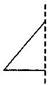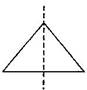Triangle (c)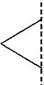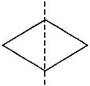Rhombus (d)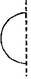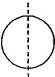Circle (e)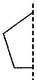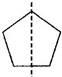Pentagon (f)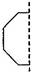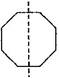Octagon

Question 4. The following figures have more than one line of symmetry. Such figures are said to have multiple lines of symmetry: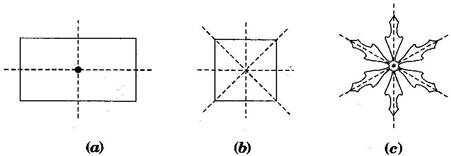Identify multiple lines of symmetry, if any, in each of the following figures:

 S.No. Problem Figures Lines of symmetry (a)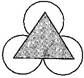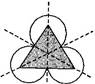(b)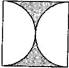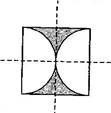(c)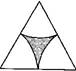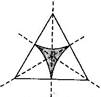(d)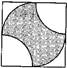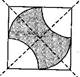(e)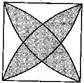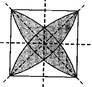(f)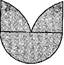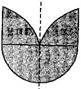(g)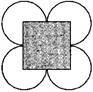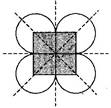(h)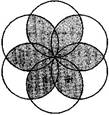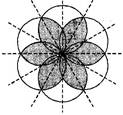Question 5. Copy the figure given here: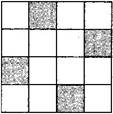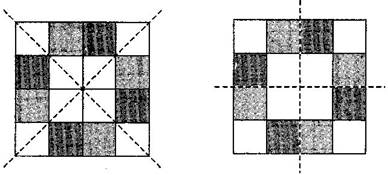Yes, there is more than one way.

Yes, this figure will be symmetric about both the diagonals.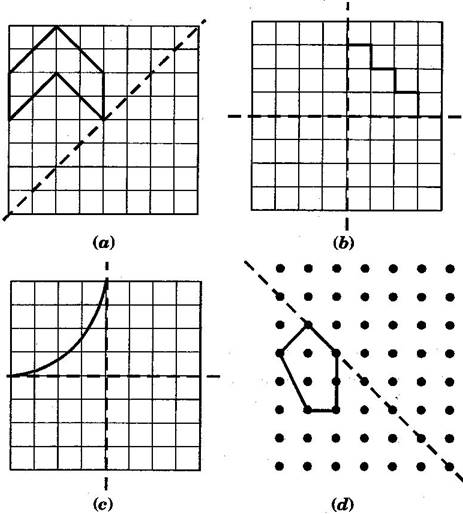Take any one diagonal as a line of symmetry and shade a few more squares to make the figure symmetric about a diagonal. Is there more than one way to do that? Will the figure be symmetric about both the diagonals?Answer: Question 6. Copy the diagram and complete each shape to be symmetric about the mirror line(s):

Question 6. Copy the diagram and complete each shape to be symmetric about the mirror line (s) :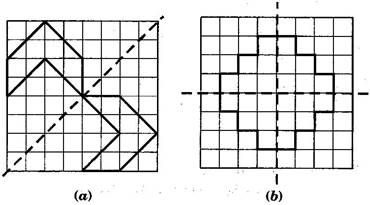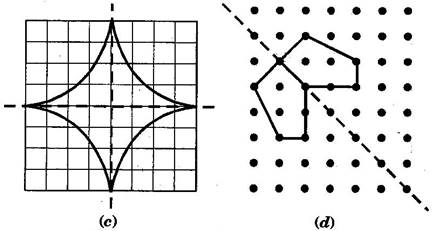Question 7. State the number of lines of symmetry for the following figures:

(a) An equilateral triangle(b) An isosceles triangle(c) A scalene triangle

(d) A square(e) A rectangle(f) A rhombus

(g) A parallelogram(h) A quadrilateral(i) A regular hexagon

(j) A circle

 S.No. Figure’s name Diagram with symmetry Number of lines (a) Equilateral triangle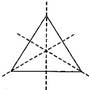3 (b) Isosceles triangle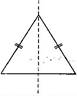1 (c) Scalene triangle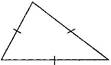0 (d) Square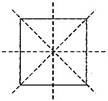4 (e) Rectangle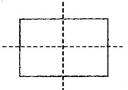2 (f) Rhombus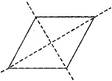2 (g) Parallelogram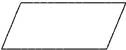0 (h) Quadrilateral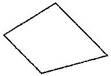0 (i) Regular Hexagon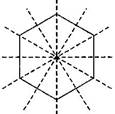6 (j) Circle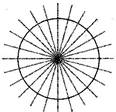Infinite

Question 8. What letters of the English alphabet have reflectional symmetry (i.e., symmetry related to mirror reflection) about:

1. a vertical mirror
2.
a horizontal mirror
3.
both horizontal and vertical mirrors

Answer: (a) Vertical mirror – A, H, I, M, O, T, U, V, W, X and Y mirror mirror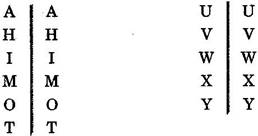(b) Horizontal mirror – B, C, D, E, H, I, O and X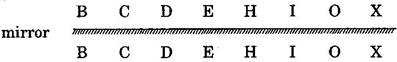(c) Both horizontal and vertical mirror – H, I, O and X

Question 9. Give three examples of shapes with no line of symmetry.